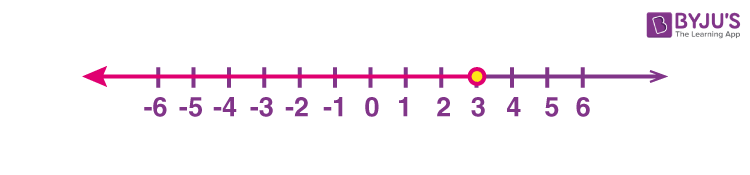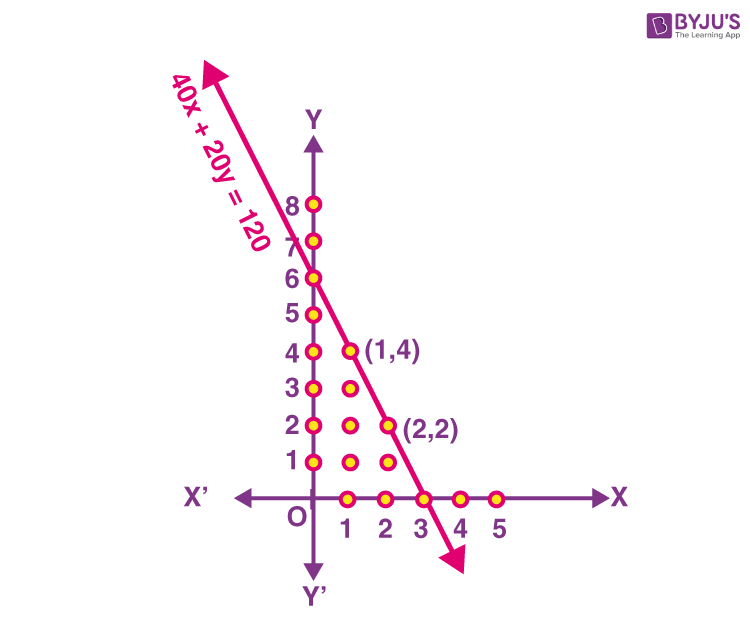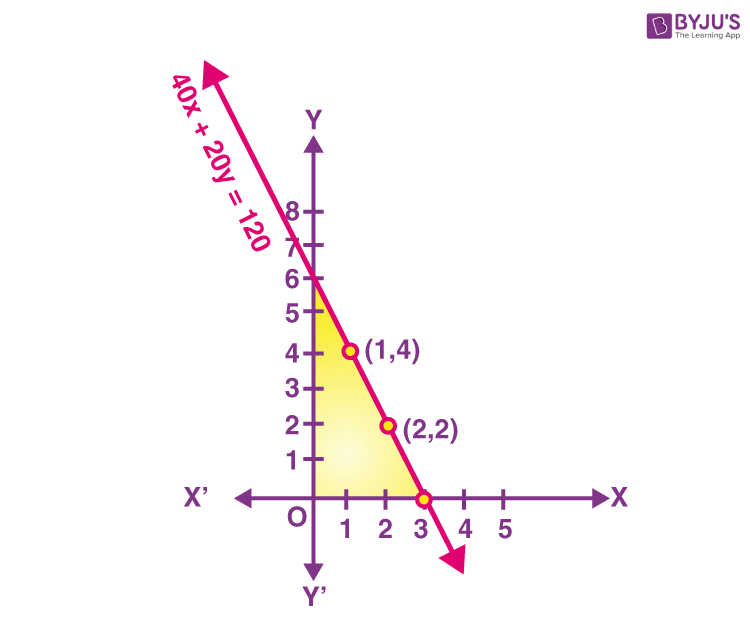Win up to 100% scholarship on Aakash BYJU'S JEE/NEET courses with ABNAT Win up to 100% scholarship on Aakash BYJU'S JEE/NEET courses with ABNAT

# Inequalities

In Mathematics, the relationship between two values that are not equal is defined by inequalities. Inequality means not equal. Generally, if two values are not equal, we use the “not equal symbol (≠)”. But to compare the values, whether it is less than or greater than, different inequalities are used. In this article, we will discuss the inequalities used in algebra, different inequality symbols, properties, and the procedure for solving the linear inequalities in one variable and two variables, with examples.

• Definition
• Symbol
• Properties
• Transitive Property
• Multiplication and Division Property
• Converse Property
• Solving Inequalities
• Linear Inequalities in One Variable
• Linear Inequalities in Two Variables
• Graphing Inequalities
• Practice Problems
• FAQs

## Inequalities Definition

In Algebra, inequality is a mathematical statement that shows the relation between two expressions using the inequality symbol. The expressions on both sides of an inequality sign are not equal. It means that the expression on the left-hand side should be greater than or less than the expression on the right-hand side or vice versa. If the relationship between two algebraic expressions is defined using the inequality symbols, then it is called literal inequalities.

Definition:If two real numbers or the algebraic expressions are related by the symbols “>”, “<”, “≥”, “≤”, then the relation is called an inequality.”

For example, x>3 (x should be greater than 3)

Open Sentence: The inequality is said to be an open sentence if it has only one variable.

For example, x < 6 (x is less than 6)

Double Inequalities: The inequality is said to be a double inequality if the statement shows the double relation of the expressions or the numbers.

Example: 3≤x<8 ( x is greater than or equal to 3 and less than 8)

## Inequality Symbols

The most familiar inequality sign is the “not equal sign (≠)”. But to compare the values on the inequalities, the following symbols are used.

Strict Inequality

The strict inequality symbols are less than symbol (<) and greater than symbol (>). These two symbols are called strict inequalities, as it shows the numbers are strictly greater than or less than each other.

For example,

• 5 < 9 ( 5 is strictly less than 9)
• 10 > 7 (10 is strictly greater than 7)

Slack Inequality

The slack inequalities are less than or equal to symbol (≤) and greater than or equal to symbol (≥). The slack inequalities represent the relation between two inequalities that are not strict.

For example,

• x ≥ 15 ( x is greater than or equal to 15)
• x ≤ 9 (x is less than or equal to 9)

## Properties of Inequalities

The following are the properties of the inequalities:

### Transitive Property

The relation between the three numbers is defined using the transitive property.

If a, b and c are the three numbers, then

If a ≥ b, and b ≥ c, then a ≥ c

Similarly,

If a ≤ b, and b ≤ c, then a ≤ c

In the above-mentioned example, if one relation is defined by strict inequality, then the result should also be in strict inequality.

For example,

If a ≥ b, and b > c, then a > c.

The addition and subtraction property of inequalities states that adding or subtracting the same constant on both sides of inequalities are equivalent to each other.

Let “m” be constant,

If x ≤ y, then x +m ≤ y+m

If x ≥ y, then x +m ≥ y+m

Similarly, for the subtraction operation,

If x ≤ y, then x -m ≤ y-m

If x ≥ y, then x – m ≥ y-m

### Multiplication and Division Property

If a positive constant number is multiplied or divided by both sides of an inequality, the inequality remains the same. But, if inequality is multiplied or divided by the negative constant number, the inequality expression will get reversed.

Let “m” be a positive constant,

If x ≤ y, then xm ≤ ym (if m>0)

If x ≥ y, then xm ≥ ym (if m>0

Let “m” be a negative constant number,

If x ≤ y, then xm ≥ ym (if m<0)

If x ≥ y, then xm ≤ ym (if m<0)

The above condition holds true for the division operation.

### Converse Property

The converse property states that if we flip the number, we have to flip the inequality symbol also.

i.e., If a ≥ b, then b ≤ a

Likewise, if a ≤ b, then b ≥ a.

## Solving Inequalities

Solving inequalities is very much similar to solving an equation. While solving the inequalities, follow the rules provided below, which do not affect the inequality direction:

• Add or subtract the same number on both sides of an inequality.
• Multiply or divide the inequality by the same positive number.
• Simplify a side of the inequality.

Now, let us discuss how to solve the linear inequalities in one variable and two variables.

### Solving Linear Inequalities in One Variable

If the linear inequality contains only one variable, then it is called linear inequality in one variable. So, here we need to find the value of the unknown variable.

Inequalities Example 1:

Solve the linear inequality in one variable: 7x+3<5x+9

Solution:

Given inequality is 7x+3<5x+9.

Subtract 5x on both sides of the inequality

Thus,

⇒ 7x+3-5x < 5x+9-5x

⇒2x+3 <9

⇒2x < 9-3

⇒ 2x < 6

⇒ x < 3

Hence, the simplified form of the linear inequality 7x+3 < 5x+9 is x < 3.

### Solving Linear Inequalities in Two Variables

If the linear inequality contains two variables, then it is called linear inequality in two variables. Here, we have to find the solution set for the pair of values of x and y, i.e., (x, y).

Inequalities Example 2:

Solve the linear inequality in two variables: 40x+20y ≤ 120

Solution:

Given inequality is 40x+20y ≤ 120 ….(1)

First, take the L.H.S of an equation, 40x+20y

Let x=0, we get

40x+20y = 40(0)+20y

= 20y

Hence, 20y ≤ 120

y ≤ 6 … (2)

Thus, if x=0, then y can take the values 0, 1, 2, 3, 4, 5, 6.

i.e., (0, 0), (0, 1), (0, 2), (0, 3), (0, 4), (0, 5), (0,6)

Similarly, if we take x = 1, 2, and 3, the possible solutions are:

(1, 0), (1, 1), (1, 2), (1, 3), (1, 4), (2, 0), (2, 1), (2, 2), (3, 0).

### Graphing Inequalities

While graphing the linear inequality, first, solve for the variable. Then, find the solution set, which will help to graph the given inequality. Now, let us learn how to graph the linear inequality in one variable and two variables.

Graphing Linear Inequality in One Variable

If the linear inequality has only one variable, then the graph can be drawn using the number line. Consider example 1 provided above,

i.e., 7x+3<5x+9

The solution set for the inequality is x <3, which is equal to (-∞, 3)

Thus, it can be drawn using the number line.Graphing Linear Inequality in Two Variables

The graph of the linear inequality in two variables should be on the cartesian plane or xy coordinate plane.

Consider an inequality as discussed above:

40x+20y ≤ 120

To graphically represent this equation, first, consider the equation 40x+20y = 120 and draw its graph.

Now, plot the points (0, 0), (0, 1), (0, 2), (0, 3), (0, 4), (0, 5), (0,6), (1, 0), (1, 1), (1, 2), (1, 3), (1, 4), (2, 0), (2, 1), (2, 2), (3, 0) in the coordinate plane.To draw the graph of inequality, take one point, say (0, 0) and check whether the point will satisfy the inequality, and we observe that the point (0, 0) satisfies the equation. Hence, plot the remaining points in the plane, and we get the inequality graph as follows:### Practice Problems

Solve the following inequality problems:

1. Solve the inequality 4x+5 < 6x+9
2. Draw the graph for inequality x≤5.
3. Solve the inequality y ≤ x+2 and represent it graphically.

## AM, GM & HM InequalityQ1

### What is meant by inequality?

If two real numbers or algebraic expressions are related using the symbols >, <, ≥, ≤, then the relation is called an inequality.

Q2

### What are the different inequality symbols?

The different inequalities in Maths are as follows:

Not equal (≠), Strict Inequalities (>, <), and Slack Inequalities (≥, ≤).

Q3

### Mention the properties of inequalities.

The properties of inequalities are listed below:
Multiplication and division property
Transitive property
Converse property.

Q4

### Mention the rules for solving inequalities.

The following rules do not affect the direction of inequality:
Add or subtract the same number on both sides of the inequality
Multiply or divide the same positive number on both sides of the inequality
Simplify a side of the equation if possible.

Q5

### Does swapping the values of the left and right-hand sides change the direction of inequality?

Yes, swapping the values of the left and right-hand sides changes the direction of the inequality.

Q6

### Give an example of a linear inequality in one variable.

x+1> 3 is an example of a linear inequality in one variable.

Q7

### What do you mean by a linear inequality in two variables?

If a linear inequality contains two variables, then it is called linear inequality in two variables. For example, 2x + 3y > 5. Here, x and y are the variables.

Q8

### What is the rule to be followed when an inequality is multiplied or divided by a negative number?

When we multiply or divide an inequality by a negative number, we should flip the inequality symbol.